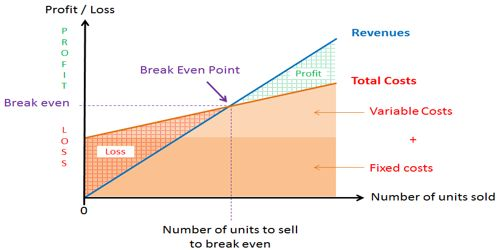Accounting

# Ways of Lowering the Break-Even PointWays of Lowering the Break-Even Point

Break-even point is that level of operation at which sales revenues for a period are equal to the costs assigned to that period. As a result, there is no net profit or loss. It can be defined as a point where total costs (expenses) and total sales (revenue) are equal. Another important usage of the break-even point is that it is helpful in recognizing the relevance of fixed and variable cost.

There are three methods is used to determined break-even analysis. These are as follows –

The equation method: The equation method uses some variation of the equation, sales = variable expenses + FC + profit; where profit is zero at the break-even point. The equation is solved to determine the break-even point in units or dollar sales.

The contribution margin method: The contribution margin method, the total fixed cost is divided by the contribution margin per unit to obtain the break-even point in units.

The graphical method: In the graphical method, total cost and total revenue data are plotted on a graph. The intersection of the total cost and the total revenue lines indicates the break-even point. The graph shows the break-even point in both units and dollars of sales.